# Symmetrical Fault Analysis

## ZBUS Formulation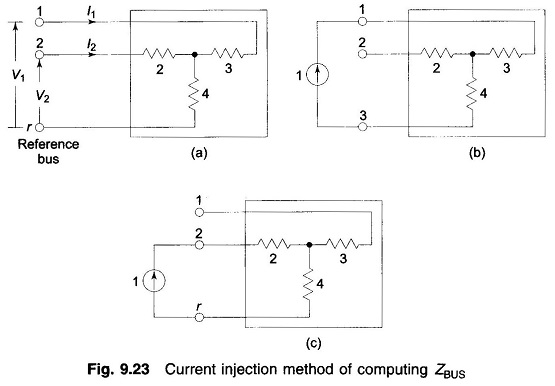ZBUS Formulation: ZBUS Formulation is given by By Inventing YBUS The sparsity of YBUS may be retained by using an efficient inversion technique and nodal impedance matrix can then be calculated directly from the factorized admittance matrix. Current Injection Method: Equation (9.33) can be written in the expanded form It immediately follows from Eq. (9.34) …

## Algorithm for Short Circuit Computation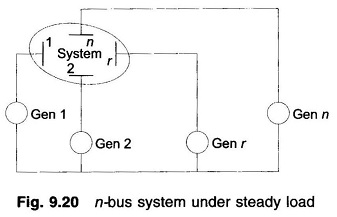Algorithm for Short Circuit Computation: So far we have carried out short circuit calculations for simple systems whose passive networks can be easily reduced. In this section we extend our study to large systems. In order to apply the four steps of Algorithm for Short Circuit Computation developed earlier to large systems, it is necessary …

## Selection of Circuit Breaker

Selection of Circuit Breaker: There are two types of Selection of Circuit Breaker ratings which require the computation of SC current are: (i) rated momentary current and (ii) rated symmetrical interrupting current. Symmetrical SC current is obtained by using subtransient reactances for synchronous machines. Momentary current (rms) is then calculated by multiplying the symmetrical momentary …

## Short Circuit Current Computation through Thevenin Theorem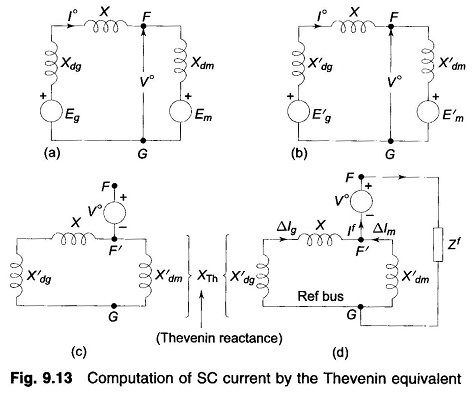Short Circuit Current Computation through Thevenin Theorem: An alternate method of computing Short Circuit Current Computation is through the application of the Thevenin theorem. This Short Circuit Current Computation method is faster and easily adopted to systematic computation for large networks. While the method is perfectly general, it is illustrated here through a simple example. …

## Short Circuit Current of Loaded Synchronous Machine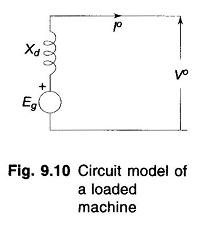Short Circuit Current of Loaded Synchronous Machine: In the previous article on the Short Circuit of a Synchronous Machine, it was assumed that the machine was operating at no load prior to the occurrence of short circuit. The analysis of Short Circuit Current of Loaded Synchronous Machine is complicated. We shall, however, present here the …

## Short Circuit of a Synchronous Machine on No Load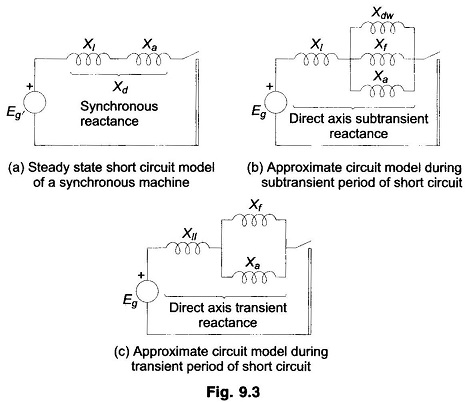Short Circuit of a Synchronous Machine on No Load: Short Circuit of a Synchronous Machine on No Load – Under steady state short circuit conditions, the armature reaction of a synchronous generator produces a demagnetizing flux. In terms of a circuit this effect is modelled as a reactance Xa in series with the induced emf. …

## Waveform of a Short Circuit Current on Transmission Line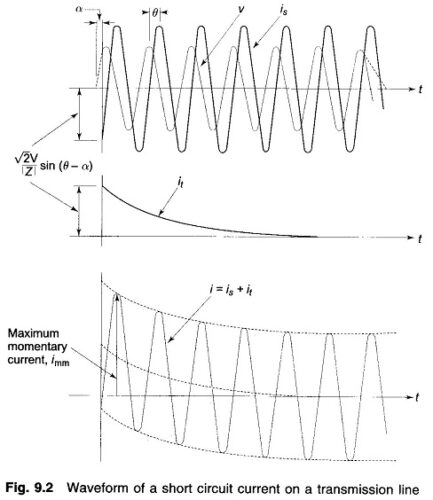Waveform of a Short Circuit Current on Transmission Line: Let us consider the Short Circuit Current on Transmission Line. Certain simplifying assumptions are made at this stage. The line is fed from a constant voltage source (the case when the line is fed from a realistic synchronous machine). Short circuit takes place when the line …

## Introduction to Symmetrical Fault in Power System

Introduction to Symmetrical Fault in Power System: Introduction to Symmetrical Fault in Power System devoted to abnormal system behavior under conditions of symmetrical short circuit (symmetrical three-phase fault). Such conditions are caused in the system accidentally through insulation failure of equipment or flashover of lines initiated by a lightning stroke or through accidental faulty operation. …

Scroll to Top